## Matrix Diagonalization

A square matrix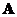is similar to a square matrix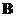if there is a non-singular matrix such that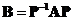. Let us call matrix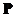as a modal matrix.

Similarity transformation has several properties:

• Identity : a matrix is similar to itself
• Commutative : ifis similar tothenis similar to.
• Transitive : ifis similar toandis similar to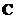, thenis similar to.
• Ifis similar tothen they have
• Equal determinant :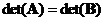• Equal rank :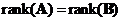• Equal trace :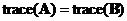• Equal transpose :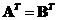• Equal inverse :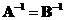• Equal matrix power :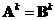• Equal matrix exponent :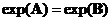Since diagonal matrix has many nice properties similar to a scalar, we would like to find matrix similarity to a diagonal matrix. The only requirement to perform similarity transformation is to find a non singular modal matrixsuch that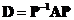. We can form modal matrixfrom the eigenvector of matrix. However, we are not sure if the modal matrixis nonsingular (has inverse).

We know that modal matrixis nonsingular when the eigenvectors of the square matrixare being linearly independent . But, again we are not sure whether the eigenvectors of the square matrixwill be linearly independent. We only know that if all the eigenvalues of the square matrixare distinct (do not have any eigenvalue of multiple values) then the eigenvectors are linearly independent. Thus, any square matrix with distinct eigenvalues can be converted into diagonal matrix by similarity transformation.

To obtain modal matrix, we perform horizontal concatenation of the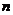linearly independent eigenvectors of matrixsuch that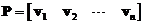. Since eigenvalues of matrixare all distinct, modal matrixhas full rank because the eigenvectors are linearly independent, therefore modal matrixhas inverse (nonsingular). The diagonal elements of diagonal matrix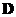consist of the eigenvalues of.

Example:

Find diagonal matrix of matrix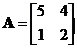Solution: First, we find the eigenvector and eigenvalues of matrix. The matrix has 2 distinct eigenvalues. Eigenvalue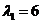has corresponding eigenvector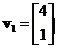and eigenvalue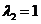has corresponding eigenvector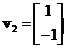. Since the eigenvalues are all distinct, the matrix is diagonalizable and the eigenvectors are linearly independent.
Next, we form modal matrix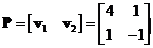. The inverse modal matrix is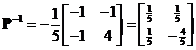.
Then, we obtain the diagonal matrix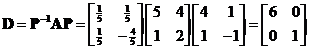. Notice that the diagonal elements are the eigenvalues. Modal matrixis not orthogonal matrix because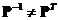.

Example:
Matrixhas eigenvalues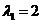(with algebraic multiplicity of 2) and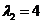(simple). The first eigenvaluehas corresponding eigenvector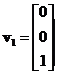and. The first eigenvaluehas geometric multiplicity of 2 because the two eigenvectors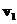and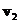are linearly independent. The second eigenvaluehas corresponding eigenvector. Since matrixhas 3 linearly independent eigenvectors, matrixis non-defective. We can form modal matrixsuch that. Notice in this example that the eigenvalues are not all distinct but the eigenvectors are linearly independent, therefore the matrix is diagonalizable.

Example:

Matrix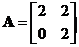has multiple eigenvalue of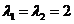. The eigenvectors associated with eigenvalues are vectors of the form of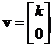for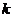any non-zero real number. Since the eigenvectors are linearly dependent, the modal matrix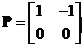has no inverse and therefore matrix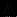is non-diagonalizable.